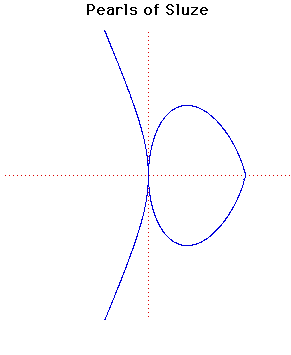# Curves

### Pearls of SluzeCartesian equation:
$y^{n} = k(a - x)^{p}x^{m}$

### Description

The curves with the equation given above, where $n, p$ and $m$ are integers, were studied by de Sluze between 1657 and 1698.

The name Pearls of Sluze was given to these curves by Blaise Pascal.

The particular curves drawn above have
$n = 4, k = 2, a = 4, p = 3, m = 2$.

### Associated Curves

Definitions of the Associated curves